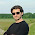10/28/2013

Two view of cam to one screen using stitching algorithm(OpenCV, example source code), (mosaic)

This source code based on ->
http://feelmare.blogspot.kr/2011/08/two-image-mosaic-paranoma-based-on-sift.html
This link page introduces how to make a mosaic image from two adjacent images.
I made two cam video to one stitching video using the source code.

After run, operate the program of 3 keys.
'q' key is quit, 'p' key is processing(stitching), 'r' is reset.

Mat TwoInOneOut(Mat Left, Mat Right);

void main()
{
VideoCapture stream1(0);   //0 is the id of video device.0 if you have only one camera
VideoCapture stream2(1);   //0 is the id of video device.0 if you have only one camera

if (!stream1.isOpened()) { //check if video device has been initialised
cout << "cannot open camera 1";
}

if (!stream2.isOpened()) { //check if video device has been initialised
cout << "cannot open camera 2";
}

// namedWindow("Processing");
// namedWindow("Left");
// namedWindow("Right");

Mat H;
int mode=0;
//unconditional loop
while (true) {
Mat cameraFrame1;
stream1.read(cameraFrame1); //get one frame form video

Mat cameraFrame2;
stream2.read(cameraFrame2); //get one frame form video

if(mode == 0)
{
imshow("Left", cameraFrame1);
imshow("Right", cameraFrame2);
}

Mat Left(cameraFrame1.rows, cameraFrame1.cols, CV_8U);
Mat Right(cameraFrame1.rows, cameraFrame1.cols, CV_8U);
cvtColor(cameraFrame1, Left, CV_RGB2GRAY, CV_8U);
cvtColor(cameraFrame2, Right, CV_RGB2GRAY, CV_8U);

if (waitKey(30) == 'p')
{
printf("Homography Matrix Processing\n");
H = TwoInOneOut(Left, Right);
mode=1;
destroyWindow("Left");
destroyWindow("Right");
}

if(waitKey(30) == 'r')
{
printf("normal mode\n");
destroyWindow("Processing");
mode=0;
}

//printf("%d %d\n", H.cols, H.rows);
if(H.cols == 3 && H.rows == 3)
{
Mat WarpImg( Left.rows*2, Left.cols*2, cameraFrame1.depth() );
//printf("%d %d\n", A.depth(), A.channels());
warpPerspective(cameraFrame2, WarpImg, H, Size(WarpImg.cols, WarpImg.rows));
Mat tempWarpImg = WarpImg(Rect(0,0,Left.cols,Left.rows));
cameraFrame1.copyTo(tempWarpImg);

/*
Mat WarpImg( Left.rows*2, Left.cols*2, CV_8U);
//printf("%d %d\n", A.depth(), A.channels());
warpPerspective(Right, WarpImg, H, Size(WarpImg.cols, WarpImg.rows));
Mat tempWarpImg = WarpImg(Rect(0,0,Left.cols,Left.rows));
Left.copyTo(tempWarpImg);
*/
if(mode ==1)
imshow("Processing", WarpImg );
//Mat t = WarpImg( Rect(0,0,B.cols, B.rows));

}

//imshow("Processing", t );

if (waitKey(30) == 'q')
break;
}

destroyAllWindows();

}

Mat TwoInOneOut(Mat Left, Mat Right)
{
Mat H;

if(Left.channels() != 1 || Right.channels() != 1)
{
printf("Channel Error\n");
return H;
}

/////////////////
//Detect the keypoints using SURF Detector
int minHessian = 300; //1500;
SurfFeatureDetector detector( minHessian );
SurfDescriptorExtractor extractor;

/////////////////
//A
std::vector< KeyPoint> kp_Left;
detector.detect( Left, kp_Left );
Mat des_Left;
extractor.compute( Left, kp_Left, des_Left );

/////////////////
//B
std::vector< KeyPoint> kp_Right;
detector.detect( Right, kp_Right );
Mat des_Right;
extractor.compute( Right, kp_Right, des_Right );

/////////////////
//Match
std::vector< vector< DMatch > > matches;
FlannBasedMatcher matcher;
matcher.knnMatch(des_Left, des_Right, matches, 2);
//matcher.knnMatch(des_Right, des_Left, matches, 2);
std::vector< DMatch > good_matches;
good_matches.reserve(matches.size());

for (size_t i = 0; i < matches.size(); ++i)
{
if (matches[i].size() < 2)
continue;

const DMatch &m1 = matches[i];
const DMatch &m2 = matches[i];

if(m1.distance <= 0.7 * m2.distance)
good_matches.push_back(m1);
}

//Draw only "good" matches
Mat img_matches;
drawMatches( Left, kp_Left, Right, kp_Right, good_matches,
img_matches, Scalar::all(-1), Scalar::all(-1),
vector< char>(), DrawMatchesFlags::NOT_DRAW_SINGLE_POINTS );
imshow("Match", img_matches);

/////////////////
//Find H
if(good_matches.size() > 20 )
{
std::vector< Point2f >  LeftMatchPT;
std::vector< Point2f >  RightMatchPT;

for( unsigned int i = 0; i < good_matches.size(); i++ )
{
//-- Get the keypoints from the good matches
LeftMatchPT.push_back( kp_Left[ good_matches[i].queryIdx ].pt );
RightMatchPT.push_back( kp_Right[ good_matches[i].trainIdx ].pt );
}

H = findHomography( RightMatchPT, LeftMatchPT, CV_RANSAC );
//H = findHomography( LeftMatchPT,RightMatchPT, CV_RANSAC );
}

return H;

}

////
The source code
-> here

1.hi , i am very interesting in this work , i used and modified it to stitching 2 video files , but i faced some problem , can you help me ,,, because i ma still beginner in this field and need to help ,

when i press "p" key for processing issue, stopped and given this massage

"Unhandled exception at 0x00007ffa68fdf8b0 in opencv.exe: 0xC0000005: Access violation reading location 0x0000000000000009."

can you help me ????

2.i was sent my problem and video files to your email,,, if you have a time ,,, can you help me to solve my problem ??
waiting your replay ..
thank you ..